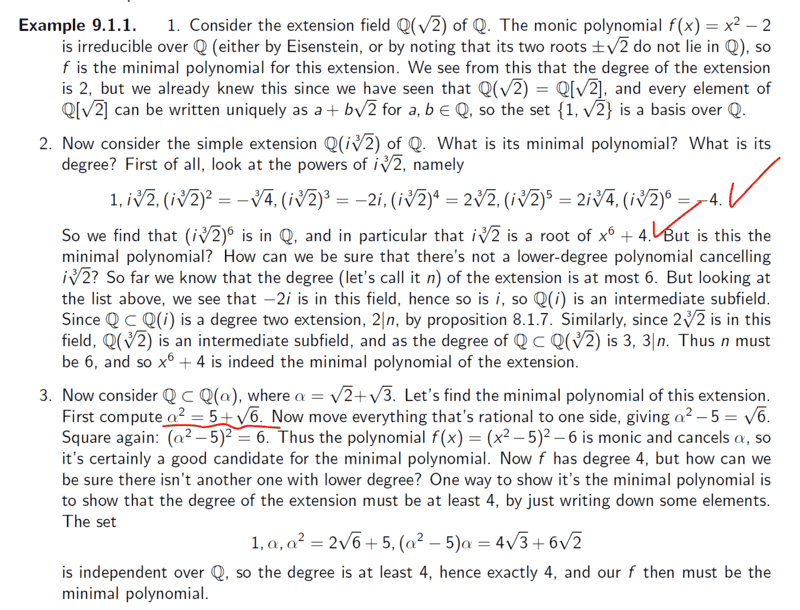# Computing the Minimal polynomial - Ring Theory

• A
• chwala
In summary, the conversation discusses a mistake in notes regarding the value of ##\alpha^2##. The correct value is ##\alpha^2=5+2\sqrt{6}##, as listed at the end. The procedure is also deemed correct. They go on to mention a text providing a proof of the irrationality of ##\sqrt{2}## using Eisenstein's theorem, but the conversation then points out that it is not necessary to use Eisenstein, as its conditions can be applied instead.

#### chwala

Gold Member
TL;DR Summary
See attached
Am going through this notes...kindly let me know if there is a mistake on highlighted part. I think it ought to be;

##α^2=5+2\sqrt{6}##chwala said:
Summary: See attached

Am going through this notes...kindly let me know if there is a mistake on highlighted part. I think it ought to be;

##α^2=5+2\sqrt{6}##

View attachment 315473
You are right. It is a mistake in the book and should be ##\alpha^2=5+2\sqrt{6}.## It is the correct value on the list at the end again. The procedure itself is correct.

Last edited:
•chwala
fresh_42 said:
You are right. It is a mistake in the book and should be ##\alpha^2=5+2\sqrt{6}.## It is the correct value on the list at the end again. The procedure it self is correct.
Thanks...let me peruse through...

The text from Chwala provides a nice, clean proof of the Irrationality of ##\sqrt 2##, though Eisenstein theorem. Per that theorem, ## x^2 -2## has no Rational solution. A nice, handwavy proof.

WWGD said:
The text from Chwala provides a nice, clean proof of the Irrationality of ##\sqrt 2##, though Eisenstein theorem. Per that theorem, ## x^2 -2## has no Rational solution. A nice, handwavy proof.
Yes, but Eisenstein uses the fact that ##\mathbb{Z}## is a UFD and ##2## is prime. With that, you don't need Eisenstein anymore:
\begin{align*}
\sqrt{2}=\dfrac{m}{n} \Longrightarrow 2n^2=m^2 \Longrightarrow 2\,|\,m \Longrightarrow 4\,|\,m^2\Longrightarrow 2\,|\,n^2
\end{align*}
contradicting the assumption that ##\dfrac{m}{n}## was cancelled.

Hence, you do not use Eisenstein, you use its conditions.